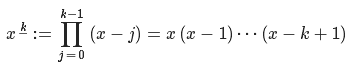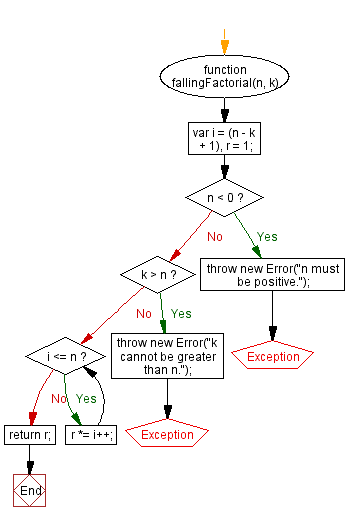# JavaScript: Calculate the falling factorial of a number

## JavaScript Math: Exercise-48 with Solution

Write a JavaScript function to calculate the falling factorial of a number.

Let x be a real number (but usually an integer).
Let k be a positive integer.
Then x to the (power of) k falling is:This is called the kth falling factorial power of x.

Sample Solution:-

HTML Code:

``````<!DOCTYPE html>
<html>
<meta charset="utf-8">
<title>JavaScript function to calculate the falling factorial of a number</title>
<body>

</body>
</html>
```
```

JavaScript Code:

``````function fallingFactorial(n, k)
{
var i = (n - k + 1),
r = 1;
if (n < 0)
{
throw new Error("n must be positive.");
}
if (k > n)
{
throw new Error("k cannot be greater than n.");
}
while (i <= n)
{
r *= i++;
}
return r;
}
console.log(fallingFactorial(10, 2));
```
```

Sample Output:

```90
```

Flowchart:Live Demo:

See the Pen javascript-math-exercise-48 by w3resource (@w3resource) on CodePen.

Improve this sample solution and post your code through Disqus

What is the difficulty level of this exercise?

Test your Programming skills with w3resource's quiz.

﻿